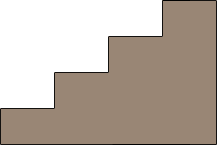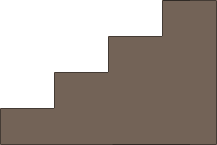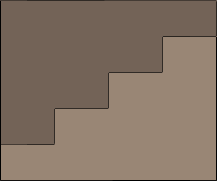SEARCH HOMEMath Central Quandaries & QueriesQuestion from jean, a student: a football stand 150 yd. long has 20 tiers of seats; each has a rise of 2 ft. and tread of 3 ft. it constructed of reinforced concrete with a cross section of 36*54. find the amount of material used in its construction.Hi Jean,

I am a little confused by your question. If there are 20 tiers of seats, each with a rise of 2 feet and a tread of 3 feet then I think the cross section will be 2 × 20 = 40 feet by 3 × 20 = 60 feet. Regardless of which it is let me show you with a smaller example how I would approach this problem.

The volume of concrete needed is the length of the stand times the area of the cross section. You know the length so you only need to find the area of the cross section. My example has 4 tiers of seats, each with a rise of 2 fet and a tread of 3 feet. Here is a diagram of the cross section.Then I drew a second diagram of the identical cross section.Finally I rotated this second diagram 180 degrees and placed it on top of the first cross section.What I have is a rectangle that is 4 × 3 = 12 feet long and 5 × 2 = 10 feet high. This rectangle has an area of 12 × 10 = 120 square feet and hence the original cross section has an area of 1/2 × 120 = 60 square feet.

I hope this helps,
HarleyMath Central is supported by the University of Regina and The Pacific Institute for the Mathematical Sciences.